In :
# %load /Users/facai/Study/book_notes/preconfig.py
%matplotlib inline

import matplotlib.pyplot as plt
import seaborn as sns

import numpy as np

import pandas as pd

from IPython.display import Image


# Chapter 6 Temporal-Difference Learning¶

DP, TD, and Monte Carlo methods all use some variation of generalized policy iteration: primarily differences in their approaches to the prediction problem.

### 6.1 TD Prediction¶

constant-$\alpha$ MC: $V(S_t) \gets V(S_t) + \alpha \underbrace{\left [ G_t - V(S_t) \right ]}_{= \sum_{k=t}^{T-1} \gamma^{k-1} \theta_k}$

\begin{align} v_\pi(s) &\doteq \mathbb{E}_\pi [ G_t \mid S_s = s] \qquad \text{Monte Carlo} \\ &= \mathbb{E}_\pi [ R_{t+1} + \gamma \color{blue}{v_\pi(S_{t+1})} \mid S_t = s ] \quad \text{DP} \end{align}

one-step TD, or TD(0): $V(S_t) \gets V(S_t) + \alpha \left [ \underbrace{R_{t+1} + \gamma \color{blue}{V(S_{t+1})} - V(S_t)}_{\text{TD error: } \theta_t} \right ]$

TD: samples the expected values and uses the current estimate $V$ instead of the true $v_\pi$.

In :
Image('./res/fig6_1.png')

Out: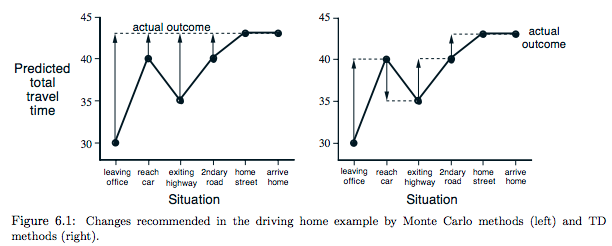In :
Image('./res/TD_0.png')

Out: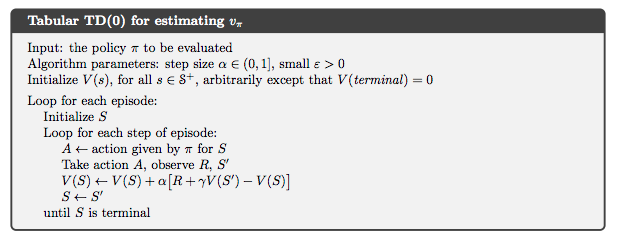### 6.2 Advantages of TD Prediction Methods¶

TD: they learn a guess from a guess - they boostrap.

1. over DP: TD do not require a model of the environment, of its reward and next-state probability distributions.
2. over Monte Carlo: TD are naturally implemented in an online, fully incremental fashion.

TD: guarantee convergence.

In practice, TD methods have usually been found that converge faster than constant-$\alpha$ MC methods on stochastic tasks.

### 6.3 Optimality of TD(0)¶

batch updating: updates are made only after processing each complete batch of training data until the value function converges.

• Batch Monte Carlo methods: always find the estimates that minimize mean-squared error on the training set.
• Batch TD(0): always find the estimates that would be exactly correct for the maximum-likelihood model of the Markov process.

### 6.4 Sarsa: On-policy TD Control¶

$Q(S_t, A_t) \gets Q(S_t, A_t) + \alpha \left [ R_{t+1} + \gamma Q(S_{t+1}, A_{t+1}) - Q(S_t,, A_t) \right ]$

In :
Image('./res/sarsa.png')

Out: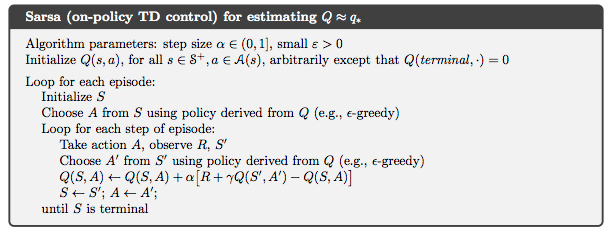### 6.5 Q-learning: Off-policy TD Control¶

$Q(S_t, A_t) \gets Q(S_t, A_t) + \alpha \left [ R_{t+1} + \gamma \color{blue}{\max_a Q(S_{t+1}, a)} - Q(S_t,, A_t) \right ]$

In :
Image('./res/q_learn_off_policy.png')

Out: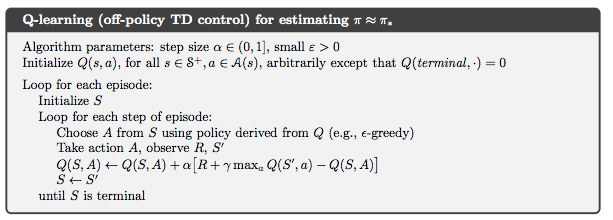### 6.6 Expected Sarsa¶

use expeteced value, how likely each action is under the current policy.

\begin{align} Q(S_t, A_t) & \gets Q(S_t, A_t) + \alpha \left [ R_{t+1} + \gamma \color{blue}{\mathbb{E}[Q(S_{t+1}, A_{t+1}) \mid S_{t+1}]} - Q(S_t,, A_t) \right ] \\ & \gets Q(S_t, A_t) + \alpha \left [ R_{t+1} + \gamma \color{blue}{\sum_a \pi(a \mid S_{t+1}) Q(S_{t+1}, a)} - Q(S_t,, A_t) \right ] \end{align}
• pro: eliminate the variance due to the random seleciton of $A_{t+1}$.

### 6.7 Maximization Bias and Double Learning¶

maximization bias:

• a maximum over estimated values => an estimate of the maximum value => significant positive bias.

root of problem: using the same samples (plays) both to determine the maximizing action and to estimate its value. => divide the plays in two sets ($Q_1, Q_2$) and use them to learn two indepedent estimates. (double learning)

$Q_1(S_t, A_t) \gets Q_1(S_t, A_t) + \alpha \left [ R_{t+1} + \gamma Q_2 \left( S_{t+1}, \operatorname{argmax}_a Q_1(S_{t+1}, a) \right) - Q_1(S_t,, A_t) \right ]$

In :
Image('./res/double_learn.png')

Out: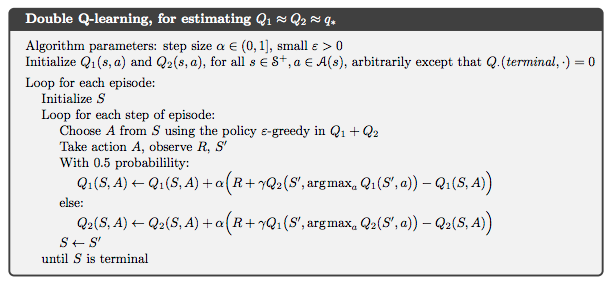### 6.8 Games, Afterstates, and Other Special Cases¶

In [ ]: